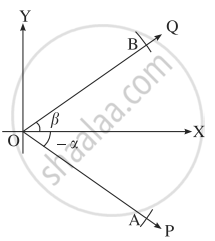# Prove, by vector method, that sin (α + β) = sin α . cos β + cos α . sin β - Mathematics and Statistics

Sum

Prove, by vector method, that sin (α + β) = sin α . cos β + cos α . sin β

#### SolutionLet ∠XOP and ∠XOQ be in standard position and m∠XOP = - α   ,m∠XOQ = β

Take a point A on ray OP and a point B on ray OQ such that OA = OB = 1.

Since cos (- α) = cos α

and sin (- α) = - sin α,

A is (cos (- α), sin (- α)),

i.e. (cos α, - sin α)

B is (cos β, sin β)

∴ bar"OA" = ("cos"  alpha)bar"i" - ("sin"  alpha).bar"j" + 0.bar"k"

bar"OB" = ("cos"  beta)bar"i" - ("sin"  beta).bar"j" + 0.bar"k"

∴ bar"OA" xx bar"OB" = |(hat"i",hat"j",hat"k"),("cos" alpha, - "sin"  alpha, 0),("cos" beta, "sin" beta, 0)|

= (cos α sin β + sin α cos β)bar"k" ....(1)

The angle between bar"OA"  "and"  bar"OB" is α + β.

Also, bar"OA", bar"OB" lie in the XY-plane.

∴ the unit vector perpendicular to bar"OA" and bar"OB" is bar"k".

∴ bar"OA" xx bar"OB" = ["OA"."OB" "sin"(alpha + beta)]bar"k"

= sin (α + β) . bar"k"`      ...(2)

∴ from (1) and (2),

sin (α + β) = sin α cos β + cos α  sin β

Concept: Vector Product of Vectors (Cross)
Is there an error in this question or solution?